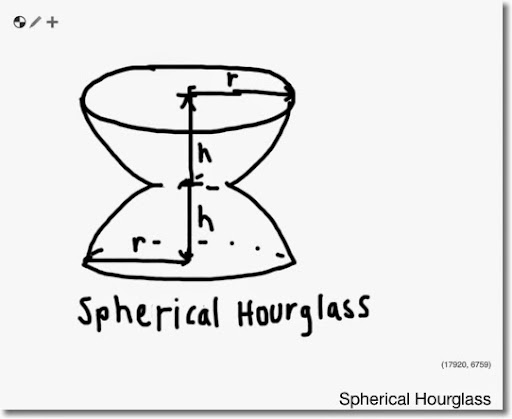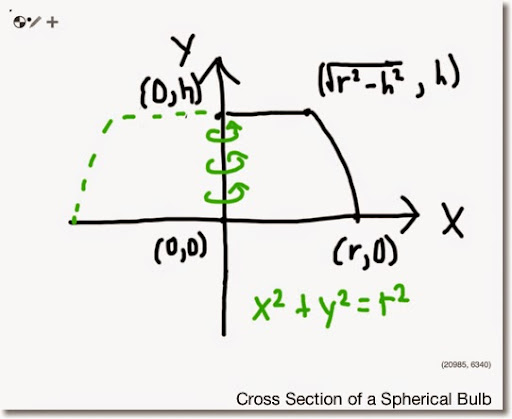## Tuesday, July 1, 2014

### Spherical HourglassLet the above picture represent a spherical hourglass, where the bulbs are sections of spheres. Assume that the two bulbs have equal size. In order to calculate the volume of a spherical hourglass, double the volume of a single spherical bulb.

A cross section of a sphere can be describe by the equation x^2 + y^2 = r^2. Surprised? A sphere is a three-dimensional circular object.By the diagram above, we calculate the volume of a spherical bulb giving radius r and height h. Using the Method of Discs with the discs rotating around the y-axis (x=0):

Top constraint: y = h
Bottom constraint: y = 0
Left constraint: x = 0
Right constraint: x = √(r^2 - y^2)

r(y) = √(r^2 - y^2)

And the volume of one of the spherical bulbs is:

h
∫ (r(y))^2 dy * π
0

h
∫ r^2 - y^2 dy * π
0

h
[ r^2 * y - y^3/3 ] * π
0

(r^2 * h - h^3/3) * π

. To get the volume of the spherical hourglass, double the volume of a spherical bulb:

V = 2 * π * (r^2 * h - h^3/3)

Note that if h = r, the bulbs are two half-spheres and that hourglass' volume:

V = 2 * π * (r^2 * r - r^3/3) = 2 * π * 2/3 * r^3 = 4/3 * π * r^3

Turns out to be the volume of a sphere.

Interesting how the mathematics checks out. With that I wish you a great day/night!

Eddie

This blog is property of Edward Shore. 2014

### Swiss Micros DM16L: Advanced Boolean and Factorial (up to 20)

Swiss Micros DM16L:   Advanced Boolean and Factorial (up to 20) Introduction The program listing, for the Swiss Micros DM16L and Hewlett Pac...